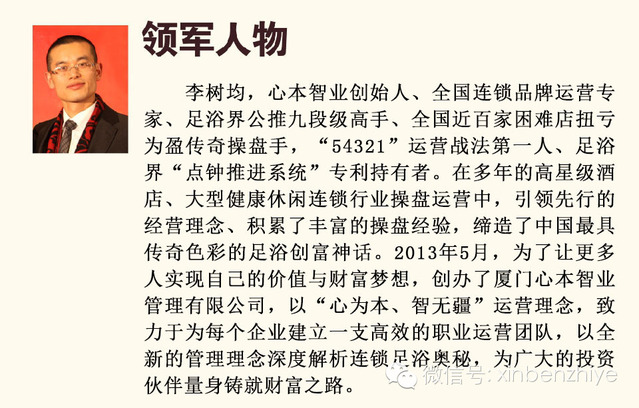theme->baseUrl; ?>/js/jquery.js"> theme->baseUrl; ?>/js/lrtk.js"> \$(document).ready(function () { \$(".rslides1_s1").html('素食珍馐'); \$(".rslides1_s2").html('文化足浴'); \$(".rslides1_s3").html('主题酒店'); \$(".rslides1_s4").html('智能软件'); \$(".rslides1_s5").html('树德公益'); });……

\$(function(){ var slide = \$('.slideplay'), ulShow = \$('.slideshow'), sLi = ulShow.find('li'), bLi = \$('.loadbar li'), len = sLi.length; var option ={ speed:3000, barWidth:142 }, barSpeed = option.speed-100; var w = sLi.first().width(), h = sLi.first().height(); var flag = 0, timer = null; ulShow.css({ width:w+'px', height:h+'px' }); slide.css({ width:w+'px', height:h+'px' }); init(); function init(){ //set slide width and height by image sLi.eq(flag).addClass('zindex').show(); bLi.eq(flag).find('em').animate({width:option.barWidth},barSpeed); timer = setTimeout(function(){ next(); },option.speed); ulShow.on('mouseover',doStop); ulShow.on('mouseleave',doAuto); bLi.on('mouseover',handPattern); bLi.on('mouseout',handOut); } function handPattern(){ doStop(); flag = \$(this).index(); imgMove(); for(i=0;i<=flag;i++) {bLi.eq(i).find('em').width(option.barWidth);} for(i=flag+1;i<=len-1;i++) {bLi.eq(i).find('em').width(0);} //bLi.find('em').css('width',0); bLi.eq(flag).find('em').width(option.barWidth); } function handOut(){ timer = setTimeout(function(){ if(flag+1 == len) {bLi.eq(flag).find('em').css('width',0);} next(); },option.speed); } function doStop(){ timer && clearTimeout(timer); bLi.eq(flag).find('em').stop(); } function doAuto(){ var em = bLi.eq(flag).find('em'), w = em.width(), leftW = option.barWidth - w , sec = (leftW * barSpeed)/option.barWidth; em.animate({width:option.barWidth},sec,function(){ if(flag==len-1){ em.width(0); next(); }else{ next(); } }); } function next(){ flag++; if(flag==len) bLi.find('em').css('width',0); flag==len && (flag=0); /*for(i=0;i<=flag;i++) {bLi.eq(i).find('em').width(option.barWidth);} for(i=flag+1;i<=len-1;i++) {bLi.eq(i).find('em').width(0);} */ doMove(); } function doMove(){ imgMove(); loadbarMove(); } function imgMove(){ sLi.eq(flag).addClass('zindex').fadeIn('slow') .siblings().removeClass('zindex').fadeOut('slow'); } function loadbarMove(){ bLi.eq(flag).find('em'). animate({width:option.barWidth},barSpeed,function(){ if(flag==len-1){ bLi.find('em').width(0); next(); }else{ next(); } }); } })